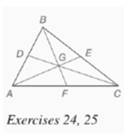Chapter 7.CR, Problem 25CR### Elementary Geometry for College St...

6th Edition
Daniel C. Alexander + 1 other
ISBN: 9781285195698

#### Solutions

Chapter
Section### Elementary Geometry for College St...

6th Edition
Daniel C. Alexander + 1 other
ISBN: 9781285195698
Textbook Problem
1 views

# Given: Δ A B C (in Exercise 24 ) with medians A E , ¯ D C , ¯ B F ¯ A G = 2 x + 2 y , G E = 2 x − y B G = 3 y + 1 , G F = x Find: B F and A ETo determine

To find: BF and AE.

Explanation

Given:

The triangle ABC with medians AE¯,CD¯,BF¯, AG=2x+2y,GE=2xy,BG=3y+1,GF=x

The point G divides the median in the ration 2:1.

Calculation:

From the figure,

AGGE=212x+2y2xy=212x+2y=4x2y2x4y=0x2y=0   ...(1)

From the figure,

BGGF=213y+1x=213y+1=2x2x3y=1

### Still sussing out bartleby?

Check out a sample textbook solution.

See a sample solution

#### The Solution to Your Study Problems

Bartleby provides explanations to thousands of textbook problems written by our experts, many with advanced degrees!

Get Started

#### Find more solutions based on key concepts